Next: Derivation of Radau equation Up: Darwin-Radau equation Previous: Calculation of gravitational potential

# Derivation and properties of Clairaut equation

Combining Equations (D.2) and (D.3) with the previous three equations, we deduce that, to first order in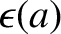, the total potential (i.e., the sum of the gravitational and centrifugal potentials) can be written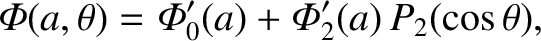(D.22)

where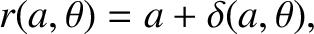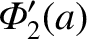(D.23)

Here, we have assumed that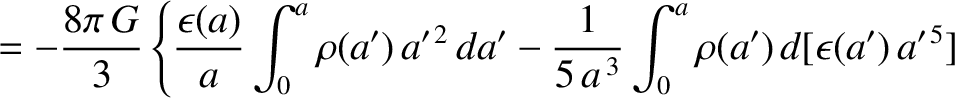. However, according to Equation (D.7), if the rotating body is in hydrostatic equilibrium (in the co-rotating frame) then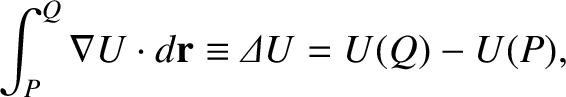is a function ofonly. In other words,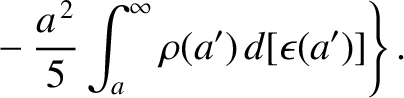, which implies that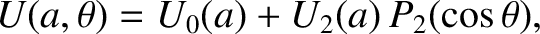(D.24)

Differentiation with respect toyields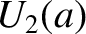(D.25)

where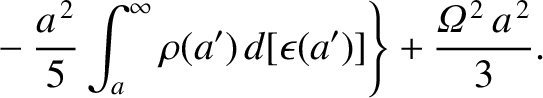(D.26)

is the mean density inside the spheroidal surface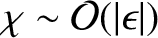. Note that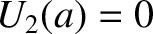(D.27)

Finally, differentiation of Equation (D.25) with respect togives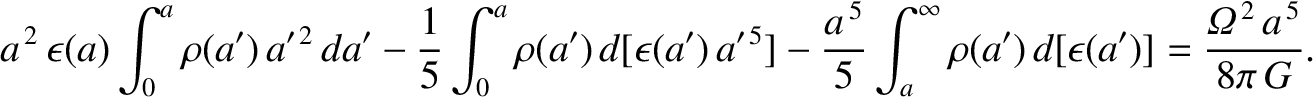(D.28)

This differential equation was first obtained by Clairaut in 1743 (Cook 1980).

Suppose that the outer boundary of the rotating body corresponds to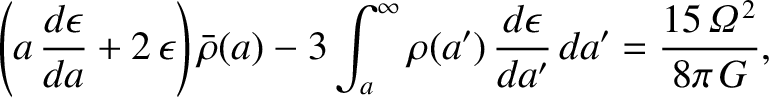, where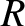is the body's mean radius. [In other words,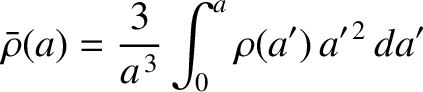for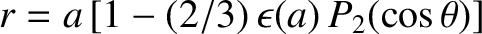.] It follows that the total mass of the body is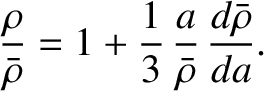(D.29)

The dimensionless parameter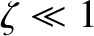, introduced in Section 6.5, is the typical ratio of the centrifugal acceleration to the gravitational acceleration at, and takes the form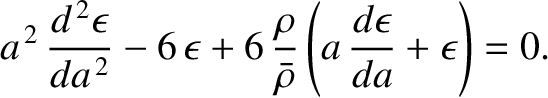(D.30)

Thus, it follows from Equation (D.25) that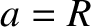(D.31)

Now, at an extremum of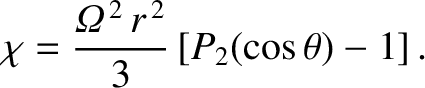, we have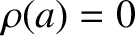. At such a point, Equation (D.28) yields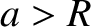(D.32)

However, if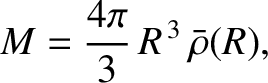is a monotonically decreasing function of, as we would generally expect to be the case, then Equation (D.27) reveals that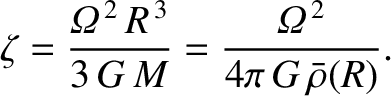. Hence, the previous equation implies that, at the extremum,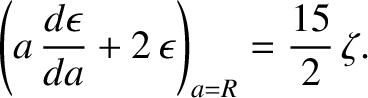has the same sign as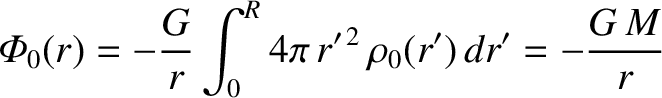. In other words, the extremum is a minimum of. This implies that it is impossible to have a maximum of. Now, a Taylor expansion of Equation (D.27) about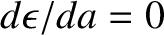, assuming that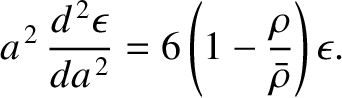, where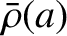, reveals that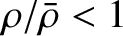is an increasing function at small. We, thus, deduce thatis a monotonically increasing function. This implies that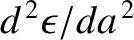has the same sign as. Hence, Equation (D.31) reveals thatis everywhere positive. In other words, ifis a monotonically decreasing function thenis necessarily a positive, monotonically increasing function. Thus, we deduce that all density contours in the body are oblate spheroids.Next: Derivation of Radau equation Up: Darwin-Radau equation Previous: Calculation of gravitational potential
Richard Fitzpatrick 2016-03-31